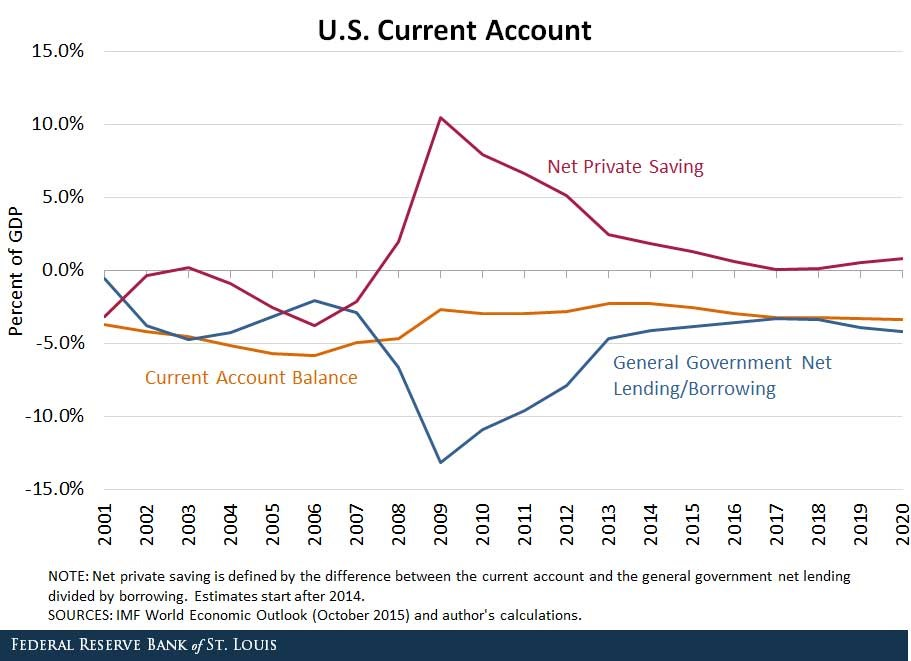# Fight Finance

#### CoursesTagsRandomAllRecentScores

Which statement is the most correct?

A stock is expected to pay the following dividends:

 Cash Flows of a Stock Time (yrs) 0 1 2 3 4 ... Dividend ($) 0 6 12 18 20 ... After year 4, the dividend will grow in perpetuity at 5% pa. The required return of the stock is 10% pa. Both the growth rate and required return are given as effective annual rates. What will be the price of the stock in 7 years (t = 7), just after the dividend at that time has been paid? Which one of the following will decrease net income (NI) but increase cash flow from assets (CFFA) in this year for a tax-paying firm, all else remaining constant? Remember: $$NI=(Rev-COGS-FC-Depr-IntExp).(1-t_c )$$ $$CFFA=NI+Depr-CapEx - ΔNWC+IntExp$$ If the USD appreciates against the AUD, the European terms quote of the AUD will or ? A risky firm will last for one period only (t=0 to 1), then it will be liquidated. So it's assets will be sold and the debt holders and equity holders will be paid out in that order. The firm has the following quantities: $V$ = Market value of assets. $E$ = Market value of (levered) equity. $D$ = Market value of zero coupon bonds. $F_1$ = Total face value of zero coupon bonds which is promised to be paid in one year.The risky corporate debt graph above contains bold labels a to e. Which of the following statements about those labels is NOT correct? Vietnamese people usually quote the Vietnamese Dong in VND per 1 USD. For example, in October 2015 the Vietnamese Dong was 22,300 VND per USD. Is this an or terms quote? If the nominal gold price is expected to increase at the same rate as inflation which is 3% pa, which of the following statements is NOT correct? The following cash flows are expected: • A perpetuity of yearly payments of$30, with the first payment in 5 years (first payment at t=5, which continues every year after that forever).
• One payment of \$100 in 6 years and 3 months (t=6.25).

What is the NPV of the cash flows if the discount rate is 10% given as an effective annual rate?

Below is a graph showing the spread or difference between government bond yields in different countries compared to the US. Assume that all governments have zero credit risk.According to the principle of cross-currency interest rate parity, which country is likely to have the greatest expected currency appreciation against the USD over the next 2 years?

Observe the below graph of the US current account surplus as a proportion of GDP.Define lending as buying (or saving or investing in) debt and equity assets.

The sum of US ‘net private saving’ plus ‘net general government lending’ equals the US: